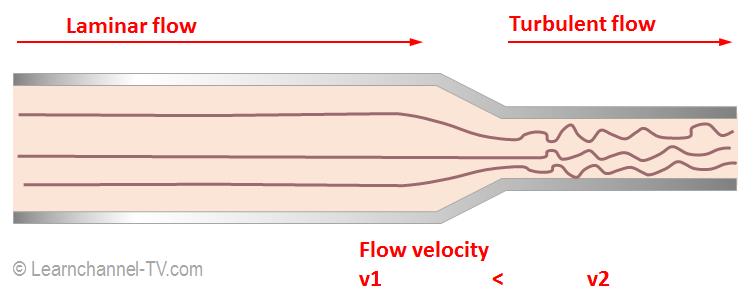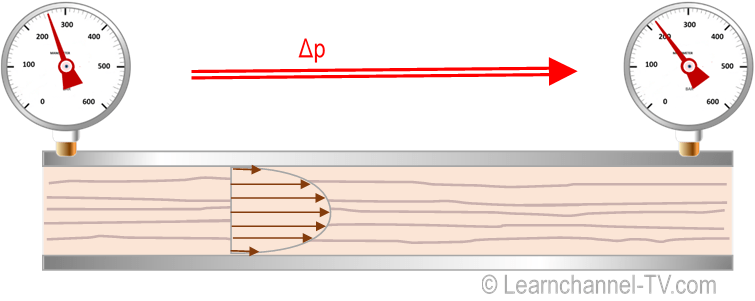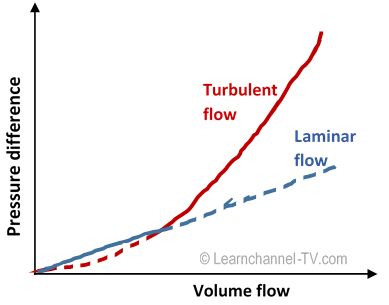# Hydraulic Resistance

## Hydraulic resistance - pressure losses on components

One problem in hydraulics is the pressure drop on pipes and components within the hydraulic circuit. Each line, each component counteracts the volume flow as soon as oil flows passe. The ratio of pressure drop to volume flow is defined as (hydraulic) resistance. The size of this resistance depends strongly on the type of pipe flow (laminar or turbulent). To be able to determine the hydraulic resistance and thus the pressure loss, we first have to take a closer look at the flow conditions:Distinction between Laminar and Turbulent Flow

Laminar flow: Laminar flow occurs when the speed of the oil flow can be regarded as slow. Here the oil moves fastest in the center of the pipe. On the pipe wall, the oil is most braked. In between, the individual oil layers slide over each other. This results in a parabolic velocity profile within the tube.
Turbulent flow: Turbulence occurs when the volume flow increases. At high flow rates, these swirls can increase such the way that the flow behaves completely chaotically.

To determine when a fluid flow turns from laminar to turbulent, the so-called The Reynolds Number (named after the physicist Osborne Reynolds) can be applied. The Reynolds number takes into account the density of the fluid, the flow rate, the viscosity and the geometry of the flow (e.g. pipe diameter).Illustration of an oil flow in a narrowing pipe cross-section

Assuming that the pipes and hoses have round and smooth inner surfaces, the hydraulic system assumes the following values ​​for the Reynolds number:  Recritical about 2200 ... 2300   and

Re <Recrit => flow is laminar
Re> Recrit => flow is turbulent

### Determine the hydraulic resistance

The Hydraulic Resistance is the resistance to flow that occurs as a result of a volume flow through hydraulic components (like pipes, valves, etc.).Pressure drop because of Hydraulic ResistanceDetermine the Hydraulic resistance

In the case of a laminar flow, the pressure difference Δp is proportional to the volume flow Q over a certain length of pipe. Thus, the flow resistance R can be easily determined:

R = Δp  / Q        Eq. 1

In a turbulent flow, the pressure difference increases quadratically with the volume flow: Δp ~ Q

For simplicity, the flow in the tube is considered to be laminar until such time as the turbulent portion has grown so far that it outweighs (intersection of both curves). From this point on, the flow changes from laminar to turbulent. If you want to determine the resistance via Eq.1, the secant applies here.